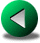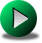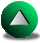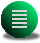Previous: The Wind-driven Circulation in
Next: The Munk Problem in
Up: Testing the Different Numerical

# Conservative Properties of the Different Numerical Formulations for a Nonlinear Problem

We compare the FE models and the discontinuous SE model to the solution given by the C-grid FD model in a test-case for which the total energy (kinetic and potential) is conserved during the time of the simulation. A geostrophically balanced eddy is initialized at the beginning of the simulation in a square domain on a beta plane approximation for all models. The shallow water equations are fully nonlinear. The fluid is inviscid, that is no eddy viscosity is applied and therefore no dynamical boundary condition is required. We introduce two versions of the LW model. The first one is the original model in which the mass matrix is lumped (see Section 2.3 for explanation) and is referred as lumped LW. The second version uses the delumped mass matrix (the full --sparse but not diagonal-- mass matrix) and is referred as delumped LW. The SE model is run on a 132 triangle mesh at nc=5. The geostrophic eddy moves slowly westward due to the sphericity of the earth and slightly southward due to the nonlinear terms (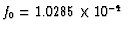s-1 andm-1s-1). The initial height is 580 m and corresponds to a velocity maximum of 1 m/s currents for a reduced gravity of g'=0.01m/s2. There is no forcing and no dissipation, therefore the total energy should be conserved. Figure 3.12 shows the results. The FD and SE models do indeed conserve energy, but all the FE models tend to lose energy. The FE models that dissipate energy moderately are the delumped LW model, closely followed by the LLS model. The HT model becomes unstable after a few days of integration and results for this model are therefore not shown. This illustrates the severe stability problem suffered by this model. The results for the lumped and delumped LW model are shown for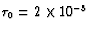s-1 (see Section 2.3 for details on). The lumped LW model appear to be more dissipative than the delumped version. We tested other values offor the lumped and delumped LW models (the results are not shown). For smallerboth versions of the model tend to be even more dissipative and the lumped version is unstable whenis too large (>10-1 s-1) or too small (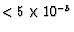s-1). The influence ofon the dynamics will be further investigated in the next test case.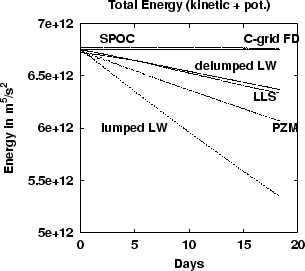Previous: The Wind-driven Circulation in
Next: The Munk Problem in
Up: Testing the Different Numerical

Frederic Dupont
2001-09-11